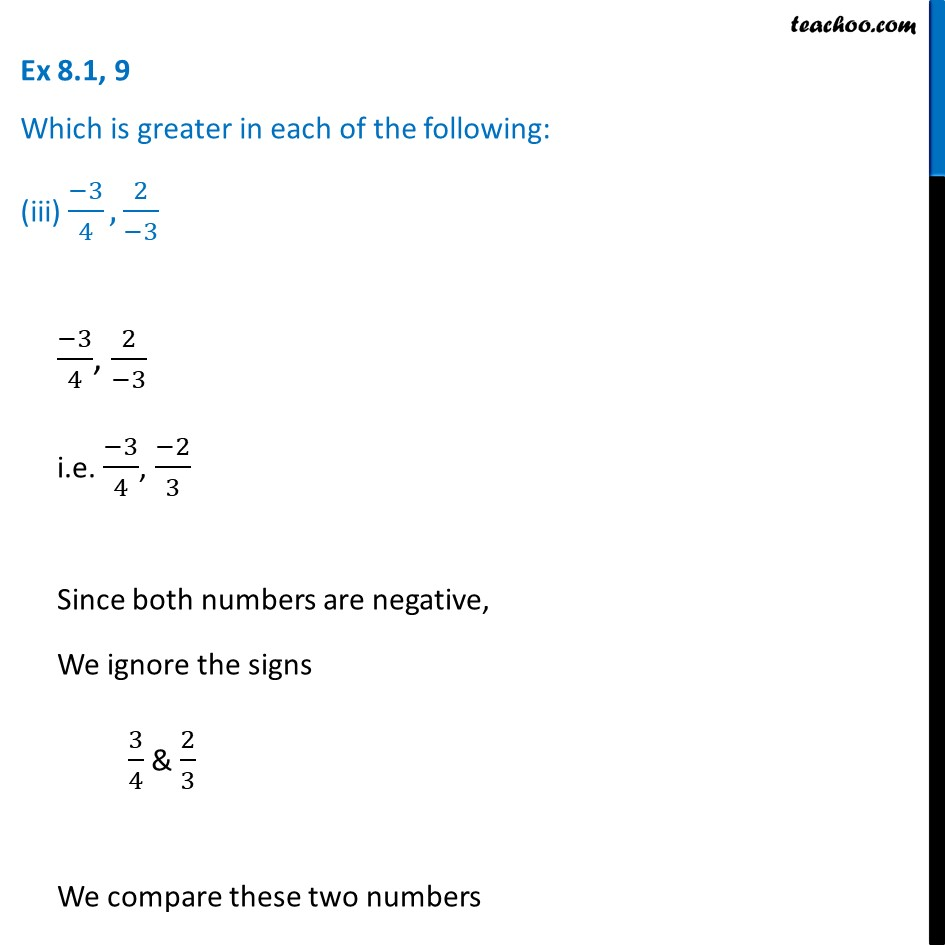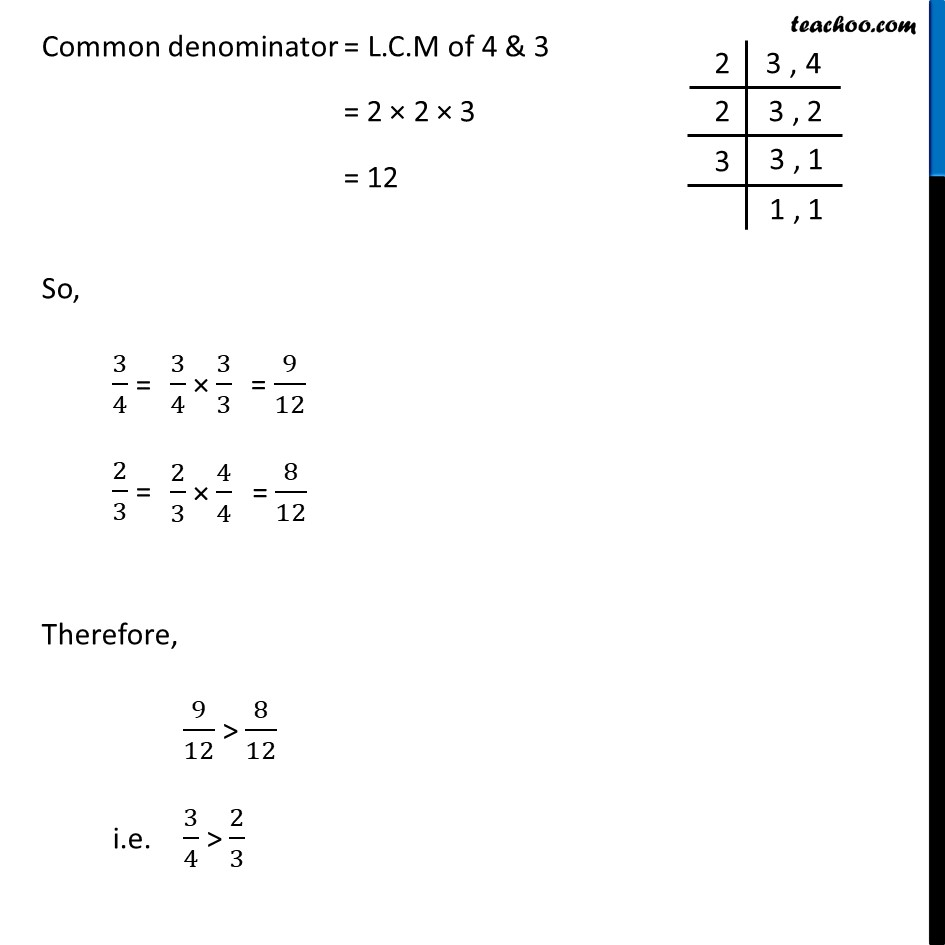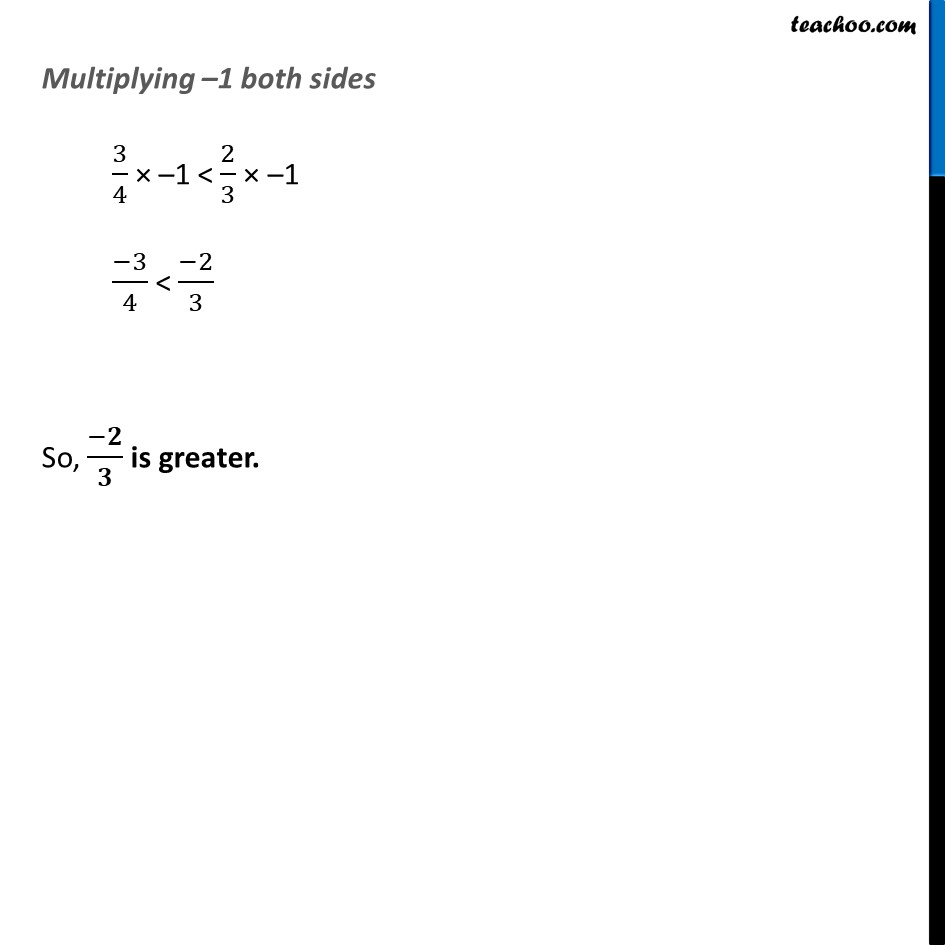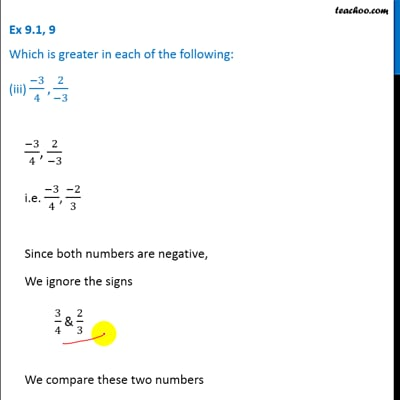Ex 8.1

Chapter 8 Class 7 Rational Numbers
Serial order wiseThis video is only available for Teachoo black users

Learn in your speed, with individual attention - Teachoo Maths 1-on-1 Class

### Transcript

Ex 8.1, 9 Which is greater in each of the following: (iii) (−3)/4, 2/(−3) (−3)/4, 2/(−3) i.e. (−3)/4, (−2)/3 Since both numbers are negative, We ignore the signs 3/4 & 2/3 We compare these two numbers Common denominator = L.C.M of 4 & 3 = 2 × 2 × 3 = 12 So, 3/4 = 2/3 = Therefore, 9/12 > 8/12 i.e. 3/4 > 2/3 Multiplying –1 both sides 3/4 × –1 < 2/3 × –1 (−3)/4 < (−2)/3 So, (−𝟐)/𝟑 is greater.